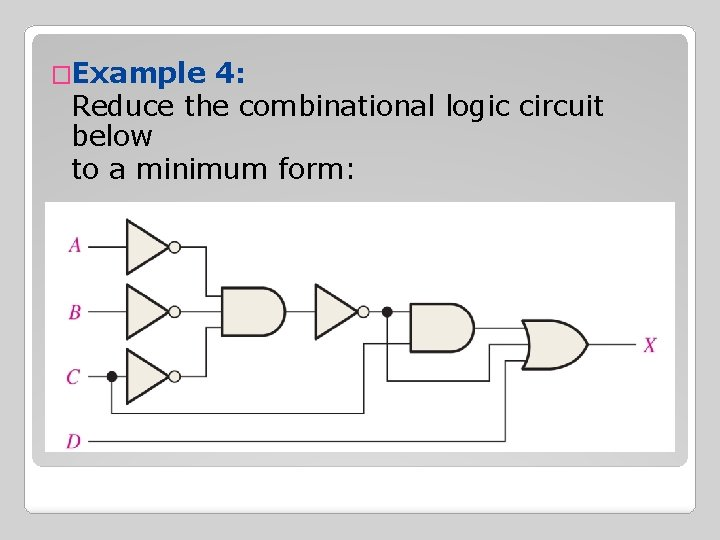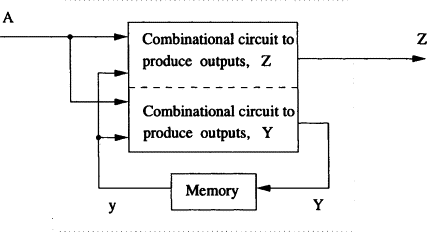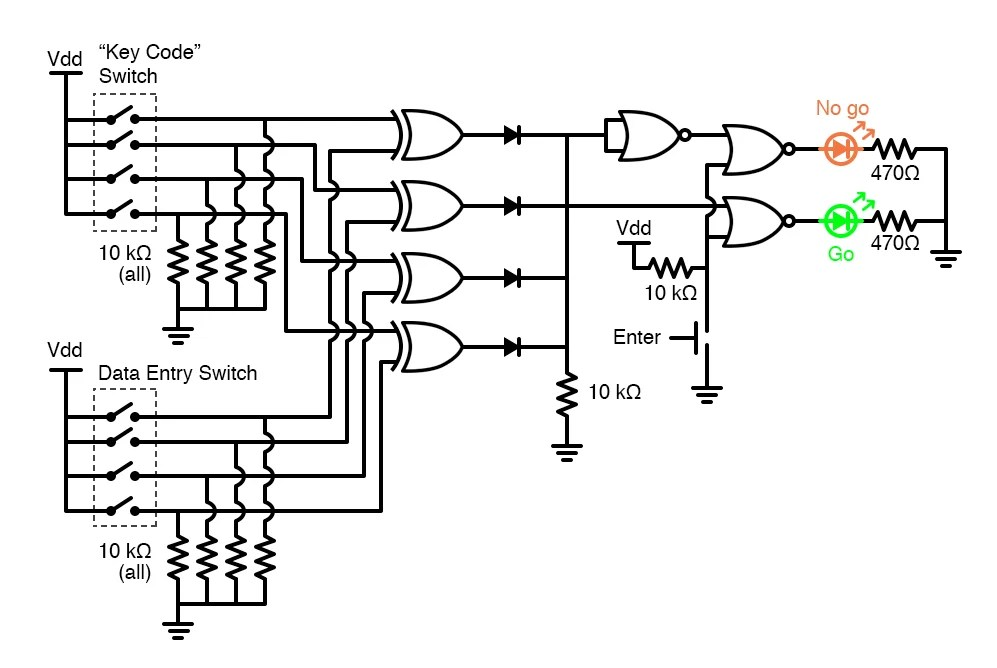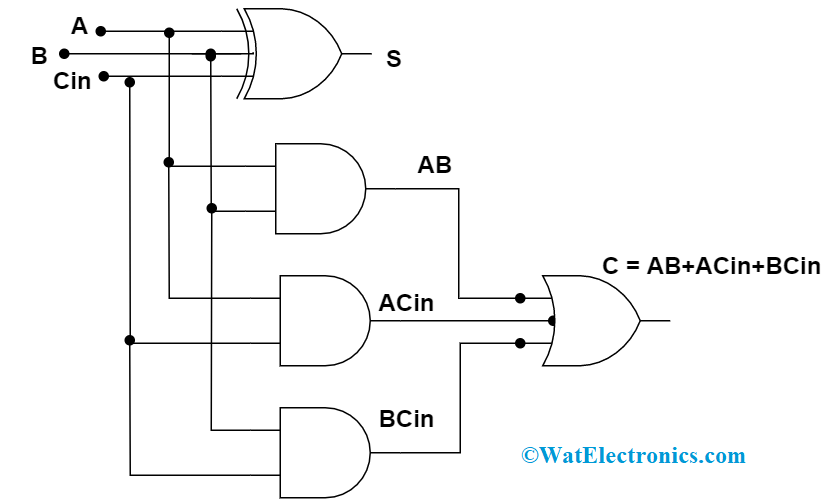# Combinational Logic Circuits Examples In Real Life

By | March 14, 2023

Combinational Logic Circuits Examples In Real Life

We often hear about the incredible power of computers and digital technology, but what about the applications of combinational logic circuits? Combinational logic circuits are found in nearly every electronic device today, from computers to smartphones and even household appliances. They’re responsible for making decisions and performing operations based on programmed inputs that can be used to control a wide variety of devices and processes.

In this article, we’ll take a closer look at examples of combinational logic circuits in real life and discuss their usefulness and importance. We’ll also cover the basics of how combinational logic works and why it’s so useful.

What is Combinational Logic?

Combinational logic is a type of digital circuit that relies on a set of logic gates. These logic gates are designed to accept input signals, process them, and produce an output. The output depends on the combination of inputs provided. They work in a binary fashion—each input is either 0 (false) or 1 (true), and the resulting output is either 0 or 1 as well.

For example, consider a simple door lock system. It has two inputs (key and handle) and one output (locked/unlocked). When both the key and the handle are in the correct position, the output will be "unlocked," allowing the user to open the door. This is a simple example of combinational logic at work.

Examples of Combinational Logic Circuits in Real Life

Computers and other digital devices rely heavily on combinational logic circuits. In fact, most modern electronics include combinational logic circuits in some form. Some examples of real-life applications include:

Computer processors: Most modern computer processors use combinational logic circuits to perform a wide range of tasks, from simple calculations to more complex operations.

Cell phones: Cell phones use combinational logic circuits to perform a variety of functions, including making calls, sending messages, and accessing the internet.

Robots: Robots use combinational logic circuits to make decisions and carry out tasks, such as navigating through a maze or finding objects.

Automatic teller machines (ATMs): ATMs use combinational logic circuits to verify user credentials, read cards, and dispense cash.

Home security systems: Home security systems use combinational logic circuits to detect intruders and trigger alarms.

Conclusion

Combinational logic circuits are a vital part of the digital world, and they are found in almost every electronic device we use today. By understanding how these circuits work, we can better understand how our digital devices work and how we can use them to our advantage.Combinational Logic Circuits JavatpointCombinational Logic Circuits Definition Examples And ApplicationsCourse S4 Computer Science Topic Unit 6 Boolean Algebra And Logic GatesChapter 5 Combinational Logic Analysis BasicThe Combinational Logic Circuit Evolved With Proposed System For Scientific DiagramCombinational Logic CircuitsCombinational Logic Circuits Definition Examples And ApplicationsAnalysis And Design Of Combinational Circuit Example Gates Truth Tables Digital Logic Lecture Slides DocsityPdf Optimization Of Combinational Logic Circuits With Genetic ProgrammingSome Common Applications Of Logic Gates Electrical4uCombinational Logic Circuits Definition Examples And ApplicationsCombinational Logic Circuit Design And Simulation Using Gates Technical ArticlesLogicblocks Experiment Guide Learn Sparkfun ComCombinatorial Circuits An Overview Sciencedirect TopicsSimple Combination Lock Digital Integrated Circuits Electronics TextbookSolved B Combination Logic Various Logical Functions Can Be Chegg ComCombinational Logic Circuits Definition Examples And ApplicationsAn Example Combinational Circuit For Implications Scientific Diagram